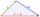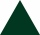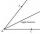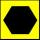# Triangle - 5th grade (10y) - math problems

#### Number of problems found: 10

• TriangleDetermine if it is possible to construct a triangle with sides 28 31 34 by calculation.
• An equilateral triangleThe perimeter of an equilateral triangle is 33cm. How long is each side?
• It is rectangular?Size of two of the angles in a triangle are: α=110°, β=40°. Is it a right triangle?
• TriangleProve whether you can construct a triangle ABC, if a=9 cm, b=6 cm, c=10 cm.
• Triangle ABCIn a triangle ABC side b measure 10 cm less than the side a and side b is half of the side c. Calculate the length of sides if the circumference of the triangle is 42 cm.
• Christmas napkinsThe girls embroidered Christmas napkins. Each napkin had the shape of a triangle with sides of 5 dm, 60 cm, and 800 mm. How many cms did the girls sew if they made 15 napkins?5 triangles + 1 square = how many sides in all
• AngleDraw angle |∠ ABC| = 130° and built its axis. What angle is between axis angle and arm of angle?
• HexagonDraw a regular hexagon inscribed in a circle with radius r=8 cm. What is its perimeter?
• TrianglesIvo wants to draw all the triangles whose two sides of which have a length of 4 cm and 9 cm, and the length of the third side is expressed in whole centimeters. How many triangles does he have?

We apologize, but in this category are not a lot of examples.
Do you have an interesting mathematical word problem that you can't solve it? Submit a math problem, and we can try to solve it.

We will send a solution to your e-mail address. Solved examples are also published here. Please enter the e-mail correctly and check whether you don't have a full mailbox.

Please do not submit problems from current active competitions such as Mathematical Olympiad, correspondence seminars etc...